Welcome to the homepage of the

Mathematical Optimization Group

 Inertial Algorithms for Non-smooth Non-convex Optimization
The inertial algorithms that we study are motivated from physics, namely from a dynamical system that describes the behaviour of a ball that is rolling down a surface subject to friction (also known as heavy-ball dynamical system). The motion of the ball accelerates due to inertia. In analogy to that, the considered algorithms generate a sequence of points that accelerates when it moves in the same direction as before. A classic method for smooth unconstrained optimization problems is the Heavy-ball method. It was introduced by Polyak in 1964 in a paper with the title "Some methods of speeding up the convergence of iteration methods" which suggests an improved performance of inertial algorithms. The global convergence of the Heavy-ball method for non-convex problems was studied by Zavriev and Kostyuk For the mechanical interpretation, we refer to

In convex optimization the heavy-ball method and its variations have led to the study of so-called accelerated schemes and optimal methods which refers to algorithms with a theoretical worst-case complexity that is of the same order as the lower complexity bound for a certain class of problems. This topic is still actively researched.

Our Contribution: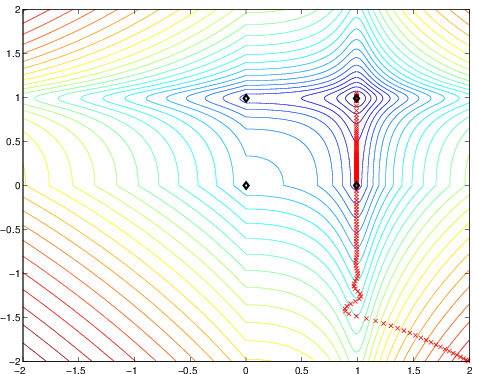P. Ochs, Y. Chen, T. Brox, T. Pock: iPiano: Inertial Proximal Algorithm for Non-convex Optimization.SIAM Journal on Imaging Sciences, 7(2):1388-1419, 2014.

We extended the applicability of the Heavy-ball method from smooth unconstrained optimization to a class of non-smooth non-convex optimization problems: problems that are the sum of a continuously differentiable function with Lipschitz continuous gradient and a non-smooth non-convex extended-valued function with a simple proximal mapping. The algorithm was termed iPiano. We proved global subsequential convergence of the algorithm to a stationary point. Moreover, this is the first work that proves global convergence of an inertial algorithm for non-smooth non-convex optimization problems. This global convergence result is holds for objective functions that satisfy the so-called Kurdyka--Lojasiewicz inequality. This is a mild assumption that is satisfied by most of the functions in practical applications. iPiano has shown state-of-the-art performance for several problems from image processing.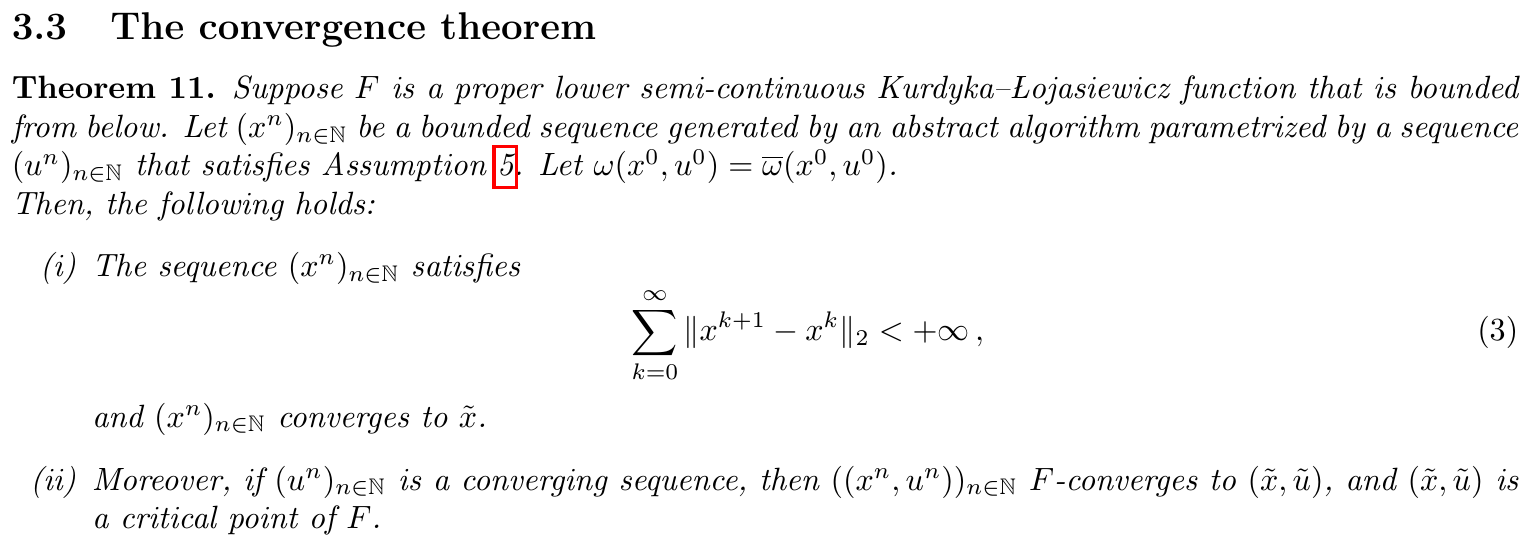P. Ochs: Unifying abstract inexact convergence theorems and block coordinate variable metric iPiano.SIAM Journal on Optimization, 29(1):541-570, 2019.

The contribution of this work is twofold: The first one is a unification of abstract convergence theorems for Kurdyka--Lojasiewicz functions. The second one uses the abstract convergence results to derive a block coordinate and variable metric variant of iPiano.P. Ochs: Local Convergence of the Heavy-ball Method and iPiano for Non-convex Optimization.Journal of Optimization Theory and Applications, 177(1):153-180, 2018.

We studied the local convergence of iPiano for a class of so-called prox-regular functions. The local convergence result shows an equivalence of iPiano to inertial alternating/averaged proximal minimization/projection methods and therefore allowed us to tranfer the convergence results to these algorithms. Key is a formula for prox-regular functions that expresses the gradient of the Moreau envelope using the proximal mapping, which is well known in the convex setting.M.C. Mukkamala, P. Ochs, T. Pock, S. Sabach: Convex-Concave Backtracking for Inertial Bregman Proximal Gradient Algorithms in Non-Convex Optimization.SIAM Journal on Mathematics of Data Science, 2(3):658-682, 2020.

The inertial algorithm with Nesterov-like extrapolation and Bregman distances proposed in this work is significant due to several reasons: (i) We introduce a novel convex concave inertial (CoCaIn) backtracking strategy. Key is the observation that the use of inertia is closely connected to certain lower bound estimates of the objective function---non-inertial methods only rely on certain simple upper bounds. Intuitively, the algorithm adapts to the "local convexity" of the objective via an efficient backtracking strategy. (ii) The algorithm shows state-of-the-art performance on several problems from Machine Learning and Computer Vision. (iii) We present the first global convergence result for an inertial algorithm with Bregman distances to a stationary point for Kurdyka--Lojasiewicz functions.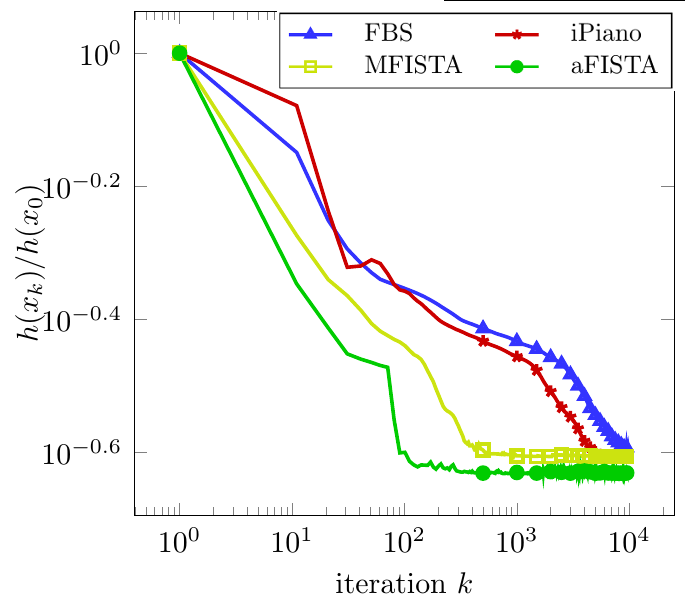P. Ochs, T. Pock: Adaptive Fista for Non-convex Optimization.SIAM Journal on Optimization, 29(4):2482-2503, 2019.

This work establishes a close connection between inertial algorithms and quasi-Newton methods. In particular, using a certain optimized extrapolation step, the SR1 quasi-Newton method can be recovered. Thanks to recent results on proximal calculus with respect to a rank-1 modified metric and the extension to proximal calculus for a rank-r modified metric, the resulting update step in Adaptive FISTA can be computed efficiently also for non-smooth non-convex additive composite problems.

The following works contribute to inertial algorithms in the convex setting.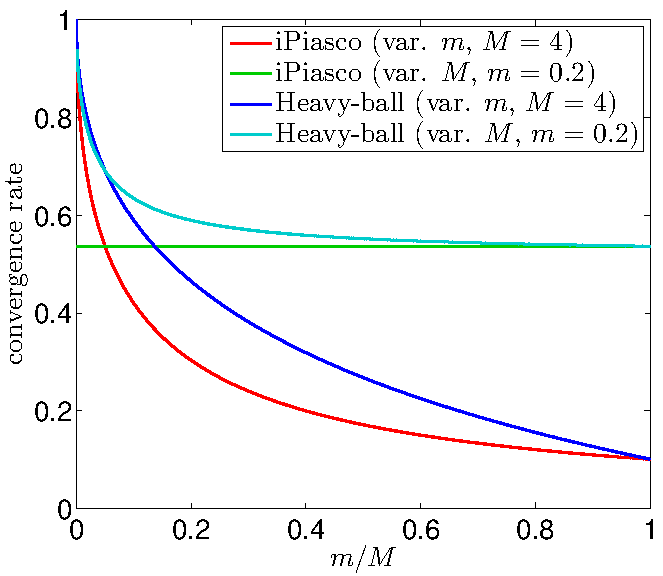P. Ochs, T. Brox, T. Pock: iPiasco: Inertial Proximal Algorithm for strongly convex Optimization.Journal of Mathematical Imaging and Vision, 53(2):171-181, 2015.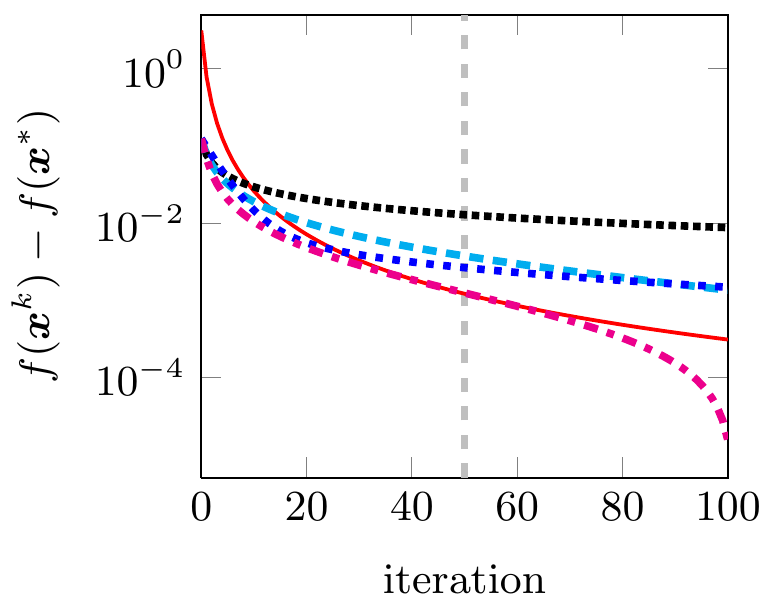D. Hafner, P. Ochs, J. Weickert, M. Reißel, S. Grewenig: FSI schemes: Fast semi-iterative solvers for PDEs and Optimisation Methods.In B. Andres, B. Rosenhahn (Eds.): German Conference on Pattern Recognition (GCPR). Lecture Notes in Computer Science, Vol. 9796, 91-102, Springer, 2016. (Best Paper Award)J.A. Tomasson, P. Ochs, J. Weickert: AFSI: Adaptive restart for fast semi-iterative schemes for convex optimisation.In T. Brox, A. Bruhn, M. Fritz (Eds.): German Conference on Pattern Recognition (GCPR). Lecture Notes in Computer Science, Vol. 11269, 669-681, Springer, 2019.

MOP Group
©2017-2020
The author is not
responsible for
the content of
external pages.